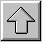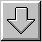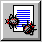# 2.2.2 简易数学

>> 1+2+3

ans =

6

>> 1*10 + 2*20 + 3*30

ans =

140

>> x=1+2+3

x =

6

>> x=1+2+3;

>> x

x =

6

>> apple=5

apples =

5

>> orange=10

orange =

10

>> total_cost=apple*2+orange*4

total_cost =

50

>> average_cost=total_cost/(apple+orange)

average_cost =

3.33334

MATLAB提供基本的算术运算有：

(+)、 (-)、 (*)、 (/)、幂次方 (^)，范例为：5+3, 5-3, 5*3, 5/3, 5^3

>> r=2;

>> area=pi*r^2;

>> area =

12.5664

>> r=2, area=pi*r^2

>> r=2; area=pi*r^2;

>> r=2;

>> area = pi ...

*r^2

>> r=2; % 键入半径

>> area=pi*r^2; % 计算面积

MATLAB可以将计算结果以不同的精确度的数字格式显示，我们可以在指令视窗上的功能选单上的Options下 选 Numerical Format，或者直接在指令视窗键入以下的各个数字显示格式的指令，以π值为例

 指令 数字值 说明 format short 3.1416 预设的 4 位有效小数位数 format long 3.14159265358979 15 位有效小数位数 format short e 3.1416e+000 4 位有效小数位数加上指数表格式